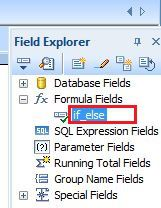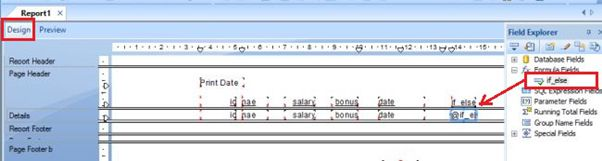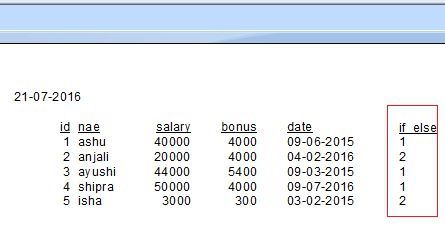# Formulas With ‘If Then Else Statement ‘

Crystal Report allows ‘If Then Else statement‘, this statement depends on the conditions. If statement finds the particular condition ‘true’, it gives an output otherwise it shows the error. This is the most basic operator of all the control statements. When we will use Boolean operators with the ‘If Then Else statement‘, if the condition is true then it allows pass the multiple conditions and returning the value in the formula.Now, First we will write the formula on the below section of formula editor and drag the data fields. Then check the error by the error checker. If the formula have no error then it shows the dialog box ‘No Error Found’.After complete, all the above process click on 'Save and Close' option in the formula editor and then go to ‘Design’ part of the report. Here, open the field explorer and drag the formula name in the design part.Then go to ‘Preview’ page of the report and see the output.Summary

Thus, we learned that 'If Else Then statement' totally depends on the conditions.
Next » Applying Calculations